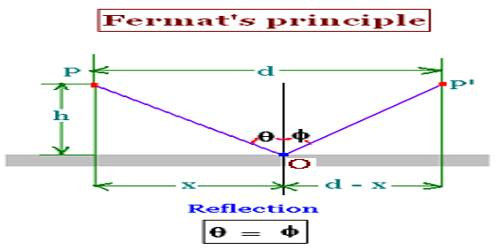# Concept of Fermat’s Principle

Fermat’s Principle – Concept

We know, a light ray starting from a point after reflection or refraction if it travels least distance to reach another point, then the time taken will also be least. So, traveling at least distance means the least time for that traveling. Now the principle relating this least distance or least time is applicable only for a plane surface. But an exception is found for a spherical surface. When reflection or refraction takes place on a spherical surface, then light rays will either travel the longest distance or least distance. But whether path traveled is the longest or least the path will always remain stationary. So Fermat’s principle is called the principle of maximum or minimum length or distance.

According to Fermat’s principle, “When light ray following laws of reflection or refraction is reflected or refracted from a plane surface, then it always follows the least path.”

From the above discussion Fermat’s principle can be expressed in general form for either plane or spherical surfaces in the following way:

The path followed by a ray of light in moving from one point to another point after any number of reflections or refractions would always be stationary.

According to the principle, a path between the object and image will be same for all the rays.

Suppose, light rays after some reflection and retraction through different media come from one point to another point. So path at the light rays will be,

S0 = μ1s1 + μ2s2 + … …. … + μsss = ΣμS = Constant

Here, μ1, μ2, μ3, … …, μn are the refractive indices at the media and s1, s2, s3 … …., sn are respectively the distance traveled in different media.

We know, differentiation of a constant is zero,

So, δ [Σμs] = 0

If, δ[f(x)] = 0, it designates the maximum value and minimum value of f(x). So, total light path (μs) will either be maximum or minimum.